Get inspired by the success stories of our students in IIT JAM MS, ISI  MStat, CMI MSc DS.  Learn More

# ISI MStat PSB 2010 Problem 2 | Combinatorics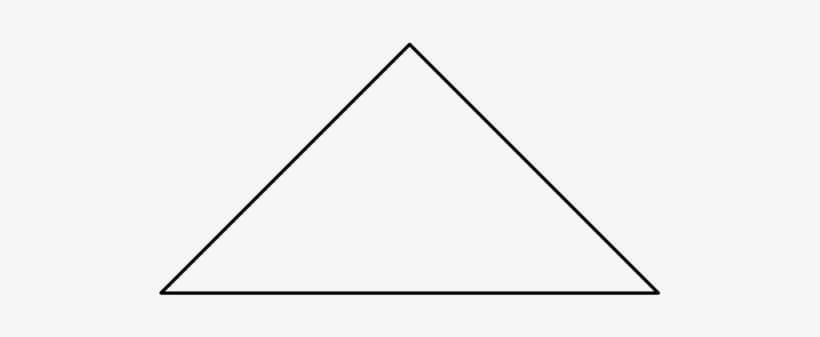This is a beautiful sample problem from ISI MStat PSB 2010 Problem 2. It's all about how many isosceles triangle with sides of integers lengths one can construct under certain conditions. We provide a detailed solution with prerequisites mentioned explicitly.

## Problem- ISI MStat PSB 2010 Problem 2

Find the total number of isosceles triangles such that the length of each side is a positive integer less than or equal to 40.

(Here equilateral triangles are also counted as isosceles triangle.)

## Prerequisites

• Basic counting principles.
• Triangle inequality.

## Solution

Let the sides of the isosceles triangle are k, k and l units, (length of the equal sides are k units)

So, our problem is all about finding triplets of form (k,k,l), where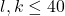. And how many such triplets can be found !

also since l,k and k are also sides of an (isosceles) triangle, so by triangle inequality,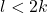. Since l is an integer so,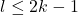So, we have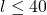and. combining we have.

now let us define,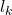: possible number of l's for a given k =1,2,....,40

here we must consider two cases.

Case-1 : when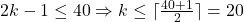So, l can be any integer between 1 and 2k-1, since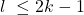when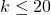so,there can be 2k-1 choices for the value of each k, when k=1,...,20.

Or,for k=1,2,....,20.

now, Case-2 : when 2k-1>40so, here l can take any integer between 1 to 40. So, there 40 choices for l for a given k, when k=21,22,...,40.

Or,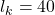for k=21,22,....,40.

Combining Case-1 and Case-2, we have,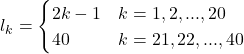So, finally, let all possible number of triplets of form (k,k,l) are =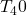So,=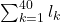=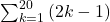+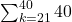=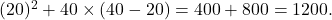So,= 1200. hence we can find 1200 such isosceles triangles with sides of integer lengths such that the length of each sides are less than or equal to 40.

## Food For Thought

Can you generalize this problem? i.e. can you find How many isosceles triangle one can construct, with sides of integer lengths, such that the sides are less than or equal to any integer N ? Can you find an elegant formula to express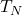, for any integer N?

## Previous ISI MStat Posts:

This is a beautiful sample problem from ISI MStat PSB 2010 Problem 2. It's all about how many isosceles triangle with sides of integers lengths one can construct under certain conditions. We provide a detailed solution with prerequisites mentioned explicitly.

## Problem- ISI MStat PSB 2010 Problem 2

Find the total number of isosceles triangles such that the length of each side is a positive integer less than or equal to 40.

(Here equilateral triangles are also counted as isosceles triangle.)

## Prerequisites

• Basic counting principles.
• Triangle inequality.

## Solution

Let the sides of the isosceles triangle are k, k and l units, (length of the equal sides are k units)

So, our problem is all about finding triplets of form (k,k,l), where. And how many such triplets can be found !

also since l,k and k are also sides of an (isosceles) triangle, so by triangle inequality,. Since l is an integer so,So, we haveand. combining we have.

now let us define,: possible number of l's for a given k =1,2,....,40

here we must consider two cases.

Case-1 : whenSo, l can be any integer between 1 and 2k-1, sincewhenso,there can be 2k-1 choices for the value of each k, when k=1,...,20.

Or,for k=1,2,....,20.

now, Case-2 : when 2k-1>40so, here l can take any integer between 1 to 40. So, there 40 choices for l for a given k, when k=21,22,...,40.

Or,for k=21,22,....,40.

Combining Case-1 and Case-2, we have,So, finally, let all possible number of triplets of form (k,k,l) are =So,==+=So,= 1200. hence we can find 1200 such isosceles triangles with sides of integer lengths such that the length of each sides are less than or equal to 40.

## Food For Thought

Can you generalize this problem? i.e. can you find How many isosceles triangle one can construct, with sides of integer lengths, such that the sides are less than or equal to any integer N ? Can you find an elegant formula to express, for any integer N?

## Previous ISI MStat Posts:

This site uses Akismet to reduce spam. Learn how your comment data is processed.

### 2 comments on “ISI MStat PSB 2010 Problem 2 | Combinatorics”

1.Arnab Chattopadhyay. says:

For general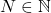we have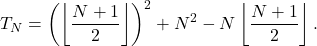1.Uttaran Chatterjee says:

yes you are correct.

### Knowledge Partner